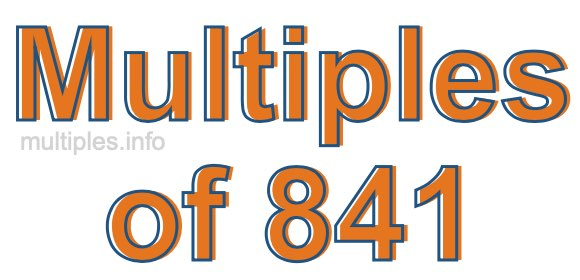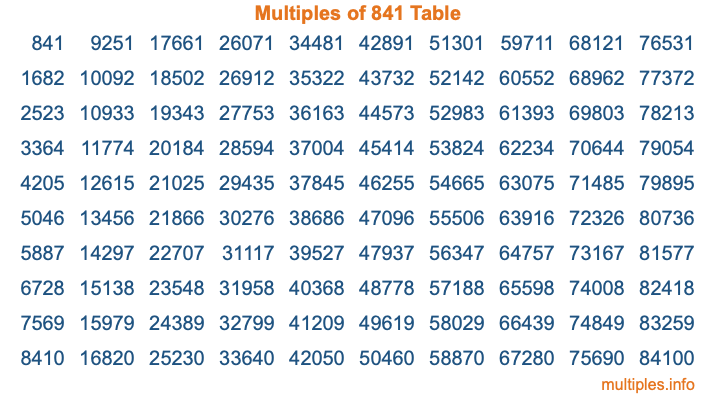Multiples of 841Welcome to the Multiples of 841 page. Here we will first teach you everything you will ever need to know about the multiples of 841, and then give you a study guide summary of everything we taught you to make sure you remember it all. Use this page to look up facts and learn information about the multiples of 841. This page will make you a multiples of eight hundred forty-one expert!

Definition of Multiples of 841
Multiples of 841 are all the numbers that when divided by 841 equal an integer. Each of the multiples of 841 are called a multiple. A multiple of 841 is created by multiplying 841 by an integer.

Therefore, to create a list of multiples of 841, you start with 1 multiplied by 841, then 2 multiplied by 841, then 3 multiplied by 841, and so on for as long as you want. Thus, the list of the first five multiples of 841 is 841, 1682, 2523, 3364, and 4205. To see a larger list of multiples of 841, see the printable image of Multiples of 841 further down on this page. We also have a category where you can choose any nth multiple of 841.

Multiples of 841 Checker
The Multiples of 841 Checker below checks to see if any number of your choice is a multiple of 841. In other words, it checks to see if there is any number (integer) that when multiplied by 841 will equal your number. To do that, we divide your number by 841. If the the quotient is an integer, then your number is a multiple of 841.

Is  a multiple of 841?

Least Common Multiple of 841 and ...
A Least Common Multiple (LCM) is the lowest multiple that two or more numbers have in common. This is also called the smallest common multiple or lowest common multiple and is useful to know when you are adding our subtracting fractions. Enter one or more numbers below (841 is already entered) to find the LCM.

Check out our LCM Calculator if you need more details about the Least Common Multiple or if you need the LCM for different numbers for adding and subtraction fractions.

nth Multiple of 841
As we stated above, 841 is the first multiple of 841, 1682 is the second multiple of 841, 2523 is the third multiple of 841, and so on. Enter a number below to find the nth multiple of 841.

th multiple of 841

Multiples of 841 vs Factors of 841
841 is a multiple of 841 and a factor of 841, but that is where the similarities end. All postive multiples of 841 are 841 or greater than 841. All positive factors of 841 are 841 or less than 841.

Below is the beginning list of multiples of 841 and the factors of 841 so you can compare:

Multiples of 841: 841, 1682, 2523, 3364, 4205, etc.

Factors of 841: 1, 29, 841

As you can see, the multiples of 841 are all the numbers that you can divide by 841 to get a whole number. The factors of 841, on the other hand, are all the whole numbers that you can multiply by another whole number to get 841.

It's also interesting to note that if a number (x) is a factor of 841, then 841 will also be a multiple of that number (x).

Multiples of 841 vs Divisors of 841
The divisors of 841 are all the integers that 841 can be divided by evenly. Below is a list of the divisors of 841.

Divisors of 841: 1, 29, 841

The interesting thing to note here is that if you take any multiple of 841 and divide it by a divisor of 841, you will see that the quotient is an integer.

Multiples of 841 Table
Below is an image of the first 100 multiples of 841 in a table. The table is in chronological order, column by column. The first column has the first ten multiples of 841, the second column has the next ten multiples of 841, and so on.The Multiples of 841 Table is also referred to as the 841 Times Table or Times Table of 841. You are welcome to print out our table for your studies.

Negative Multiples of 841
Although not often discussed or needed in math, it is worth mentioning that you can make a list of negative multiples of 841 by multiplying 841 by -1, then by -2, then by -3, and so on, to get the following list of negative multiples of 841:

-841, -1682, -2523, -3364, -4205, etc.

Multiples of 841 Summary
Below is a summary of important Multiples of 841 facts that we have discussed on this page. To retain the knowledge on this page, we recommend that you read through the summary and explain to yourself or a study partner why they hold true.

There are an infinite number of multiples of 841.

A multiple of 841 divided by 841 will equal a whole number.

841 divided by a factor of 841 equals a divisor of 841.

The nth multiple of 841 is n times 841.

The largest factor of 841 is equal to the first positive multiple of 841.

841 is a multiple of every factor of 841.

841 is a multiple of 841.

A multiple of 841 divided by a divisor of 841 equals an integer.

841 divided by a divisor of 841 equals a factor of 841.

Any integer times 841 will equal a multiple of 841.

Multiples of a Number
Here you can get the multiples of another number, all with the same attention to detail as we did for multiples of 841 on this page.

Multiples of
Multiples of 842
Did you find our page about multiples of eight hundred forty-one educational? Do you want more knowledge? Check out the multiples of the next number on our list!